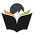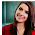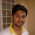# Computer System Architecture Set 18

## Questions 171 to 180

171.
Which of the following is responsible for coordinating various operations using timing signals?
 (a) Arithmetic-logic unit (b) Control unit (c) Memory unit (d) Input unit (e) Output unit.
172.
What kind of Information is stored inside the computer?
 (a) Binary form (b) ASCII code form (c) Decimal form (d) Alpha numeric (e) Numeric form.
173.
A parity bit
 (a) Is used to indicate uppercase letters (b) Is used to detect errors (c) Is the first bit in a byte (d) Is the last bit in a byte (e) Is used to indicate lowercase letters.
174.
A four input multiplexer has inputs A, B, C and D and select lines S0 and S1.  Which of the following statement(s) where the output F is given by

Which of the above statements is/are true?
 (a) Only (I) above (b) Only (II) above (c) Only (III) above (d) Both (I) and (II) above (e) Both (II) and (III) above.
175.
Consider the following Karnaugh map:
Which of the following is the most compact form of Boolean expression which corresponds to the map?
 .
176.
What do you call the given statement as?
The information which will be used in near future is likely to be in use already is”
 (a) Spatial Locality (b) Locality (c) Hit ratio (d) Temporal Locality (e) Effective access time.
177.
In which page replacement algorithm where there is a replacement of a page, which will not be used for the longest period of time?
 (a) MFU (b) LRU (c) OPT (d) FIFO (e) LFU.
178.
Which of the following is supplied by one unit to indicate to other unit when the transfer has to occur?
 (a) Strobe pulse (b) Time slice (c) Access right (d) Logic gate (e) Program.
179.
A Set of Logical Addresses as is called
 (a) Memory space (b) Disk space (c) Address space (d) Location (e) Pages.
180.
Which of the following can be used for checking errors in transmission?
 (a) Full duplex (b) Half duplex (c) CRC (d) Full adder (e) Binary adder.

29  30  31  32  33  34  35  36  37  38  39  Next >>

1.2.3.4.